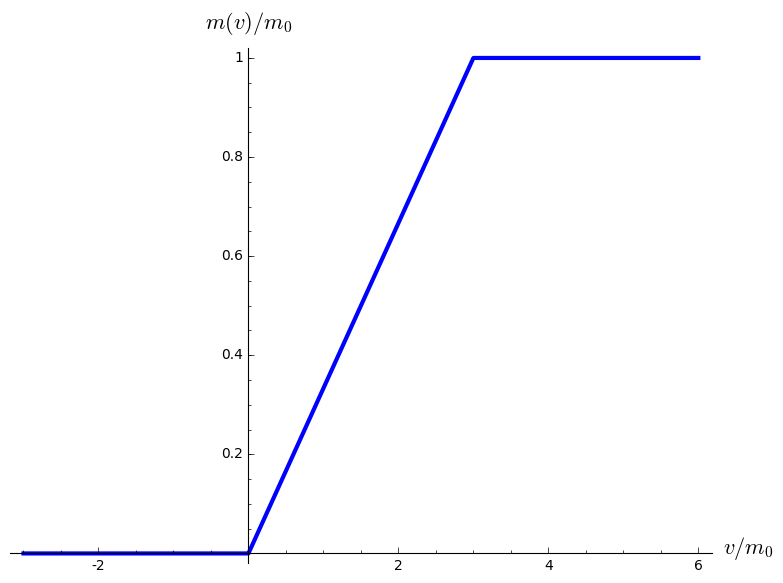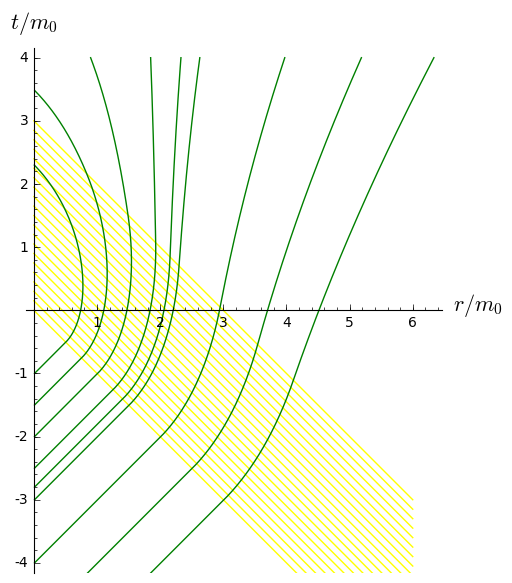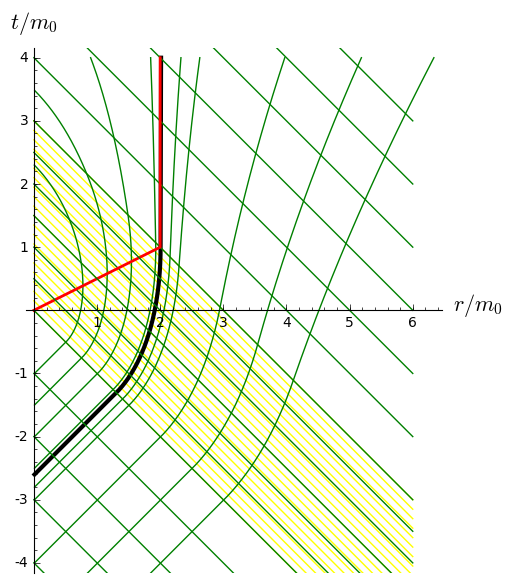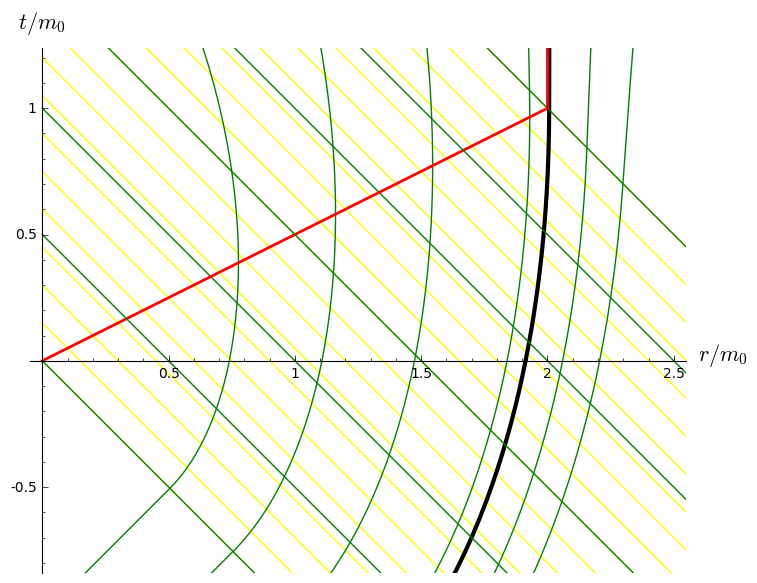# Event and trapping horizons in Vaidya spacetime¶

This Jupyter/SageMath worksheet is relative to the lectures Geometry and physics of black holes

These computations are based on SageManifolds (version 1.0, as included in SageMath 7.5 and higher versions)

Click here to download the worksheet file (ipynb format). To run it, you must start SageMath with the Jupyter notebook, with the command sage -n jupyter

NB: a version of SageMath at least equal to 7.5 is required to run this worksheet:

In :
version()

Out:
'SageMath version 8.0.beta6, Release Date: 2017-05-12'

First we set up the notebook to display mathematical objects using LaTeX formatting:

In :
%display latex


## Spacetime¶

We declare the spacetime manifold $M$:

In :
M = Manifold(4, 'M')
print(M)

4-dimensional differentiable manifold M


We use coordinates $(t,r,\theta,\phi)$ analogous to the 3+1 Eddington-Finkelstein coordinates in Schwarzschild spacetime, i.e. coordinates such that the advanced time $v = t+r$ is constant on the ingoing radial null geodesics:

In :
X.<t,r,th,ph> = M.chart(r't r:(0,+oo) th:(0,pi):\theta ph:(0,2*pi):\phi')
X

Out:
In :
X.coord_range()

Out:

## Metric tensor¶

The metric tensor corresponding to the Vaidya solution is:

In :
m = function('m')
g = M.lorentzian_metric('g')
g[0,0] = -(1-2*m(t+r)/r)
g[0,1] = 2*m(t+r)/r
g[1,1] = 1+2*m(t+r)/r
g[2,2] = r^2
g[3,3] = (r*sin(th))^2
g.display()

Out:

## Einstein equation¶

Let us compute the Ricci tensor associated with the metric $g$:

In :
Ric = g.ricci()
Ric.display()

Out:

The notation $\frac{\partial m}{\partial(r+t)}$ to denote $\frac{\mathrm{d}m}{\mathrm{d}v}$ is quite unfortunate (this shall be improved in a future version). The display of the corresponding symbolic expression is slightly better:

In :
Ric[0,0].expr()

Out:

The Ricci scalar is vanishing:

In :
g.ricci_scalar().display()

Out:

The energy-momentum vector ensuring that the Einstein equation is fulfilled is then:

In :
T = 1/(8*pi)*Ric
T.display()

Out:

Since $v=t+r$, we have $\mathrm{d}v = \mathrm{d}t + \mathrm{d}r$:

In :
dv = M.scalar_field({X: t+r}, name='v').differential()
dv.display()

Out:

The derivative of the function $m(v)$:

In :
v = var('v')
mp(v) = diff(m(v),v)
mp(v)

Out:
In :
T == 1/(4*pi)*mp(t+r)/r^2 * dv*dv

Out:

The future-directed null vector along the ingoing null geodesics:

In :
ks = -dv.up(g)
print(ks)
ks.display()

Vector field on the 4-dimensional differentiable manifold M

Out:
In :
g(ks,ks).display()

Out:

Let us consider the vector field:

In :
l = M.vector_field(name='l', latex_name=r'\ell')
l = 1
l = (r - 2*m(t+r))/(r+2*m(t+r))
l.display()

Out:

It is a null vector:

In :
g(l,l).display()

Out:

Moreover it is a pregeodesic vector field:

In :
nab = g.connection()
acc = nab(l).contract(l)
acc.display()

Out:
In :
acc/l

Out:
In :
acc/l

Out:
In :
acc/l == acc/l

Out:

## Integration of the outgoing radial null geodesics¶

The outgoing radial null geodesics are the field lines of $\ell$; they obey thus to $$\frac{\mathrm{d}r}{\mathrm{d}t} = \frac{\ell^r}{\ell^t}$$. Hence the value of $\frac{\mathrm{d}r}{\mathrm{d}t}$:

In :
drdt = (l / l).expr()
drdt

Out:

Let us choose a simple function $m(v)$:

In :
v0 = var('v0')
m0 = var('m0')
h(v) = (1+sgn(v))/2  # the Heaviside function
m1(v) = m0* (h(v)*h(v0-v)*v/v0 + h(v-v0))
m1(v)

Out:
In :
plot(m1(v).subs({m0: 1, v0: 3}), (v,-3, 6), thickness=3,
axes_labels=[r'$v/m_0$', r'$m(v)/m_0$'])

Out:We plug this function into the expression of $\frac{\mathrm{d}r}{\mathrm{d}t}$ found above:

In :
drdt1 = drdt.substitute_function(m, m1)
drdt1

Out:

and we perform a numerical integration for $v_0 = 3 m_0$

In :
drdt0 = drdt1.subs({m0: 1, v0: 3})
drdt0

Out:
In :
outgeods = []
tmax = 4
for t0 in [-6, -5, -4, -3, -2.8, -2.5, -2, -1.5, -1]:
sol = desolve_rk4(drdt0, r, ivar=t, ics=[t0,0.01], end_points=[t0, tmax], step=0.02)
outgeods.append(line([[s,s] for s in sol if s>0], color='green'))

In :
graph = Graphics()
rmax = 6
nl = 20
for i in range(nl+1):
t0 = float(i)/float(nl)*3
graph += line([(0,t0), (rmax, t0-rmax)], color='yellow')

In :
for geod in outgeods:
graph += geod
show(graph, aspect_ratio=1, ymin=-4, axes_labels=[r'$r/m_0$', r'$t/m_0$'])The event horizon:

In :
t0 = -2.6
sol = desolve_rk4(drdt0, r, ivar=t, ics=[t0,0.01], end_points=[t0, tmax], step=0.02)
hor = line([[s,s] for s in sol if s>0], color='black', thickness=3)
graph += hor

In :
ingeods = []
rmax = 6
for t0 in range(-6, 10):
ingeods.append(line([(0,t0), (rmax, t0-rmax)], color='green'))
for geod in ingeods:
graph += geod


The trapping horizon:

In :
trap = line([(0,0), (2,1), (2,tmax)], color='red', thickness=2)
graph += trap

In :
for t0 in [0.5, 1.5, 2.5]:
graph += line([(0,t0), (rmax, t0-rmax)], color='green')
show(graph, aspect_ratio=1, ymin=-4, ymax=4, axes_labels=[r'$r/m_0$', r'$t/m_0$'])In :
graph.save("vaidya.pdf", aspect_ratio=1, ymin=-4, ymax=4,
axes_labels=[r'$r/m_0$', r'$t/m_0$'])


A zoom on the trapping horizon in its dynamical part: notice that the "outgoing" null geodesics cross it with a vertical tangent, in agreement with the cross-sections of the trapping horizon being marginally trapped surfaces.

In :
show(graph, aspect_ratio=1, ymin=-0.8, ymax=1.2, xmax=2.5,
axes_labels=[r'$r/m_0$', r'$t/m_0$'])In [ ]: